How Cheenta works to ensure student success?
Explore the Back-Story

# NMTC 2015 Stage II - BHASKARA (Class 9, 10) - Problems and Solutions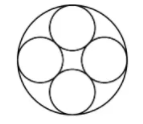###### Problem 1

a)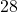integers are chosen from the interval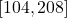. Show that there exit two of them having a common prime divisor.
b)is a line segment .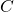is a point on.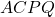andare squares drawn on the same side, Prove the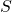is the orthocentre of the triangle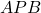.

###### Problem 2

a)are distinct real numbers such that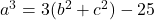,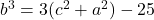,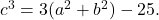Find the numerical value of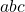.
b)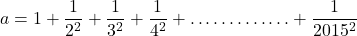find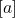, wheredenotes the integer part of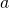.

###### Problem 3

The arithmetic mean of a number of pair wise distinct prime numbers is. Determine the biggest prime among them.

###### Problem 4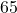bugs are placed at different squares of a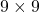square board. A bug in each moves to a horizontal or vertical adjacent square. No bug makes two horizontal or two vertical moves in succession. Show that after some moves, there will be at least two bugs in the same square.

###### Problem 5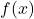is a fifth degree polynomial. It is given that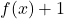in divisible by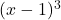and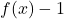is divisible by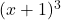. Find.

###### Problem 6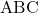and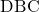are two equilateral triangles on the same base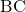.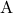point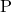is taken on the circle with centre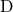, radius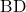. Show that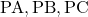are the sides of a right triangle.

###### Problem 7are real numbers such that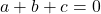and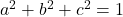. Prove that. When does the equality hold?

###### Problem 1

a)integers are chosen from the interval. Show that there exit two of them having a common prime divisor.
b)is a line segment .is a point on.andare squares drawn on the same side, Prove theis the orthocentre of the triangle.

###### Problem 2

a)are distinct real numbers such that,,Find the numerical value of.
b)find, wheredenotes the integer part of.

###### Problem 3

The arithmetic mean of a number of pair wise distinct prime numbers is. Determine the biggest prime among them.

###### Problem 4bugs are placed at different squares of asquare board. A bug in each moves to a horizontal or vertical adjacent square. No bug makes two horizontal or two vertical moves in succession. Show that after some moves, there will be at least two bugs in the same square.

###### Problem 5is a fifth degree polynomial. It is given thatin divisible byandis divisible by. Find.

###### Problem 6andare two equilateral triangles on the same base.pointis taken on the circle with centre, radius. Show thatare the sides of a right triangle.

###### Problem 7are real numbers such thatand. Prove that. When does the equality hold?

This site uses Akismet to reduce spam. Learn how your comment data is processed.

### Knowledge Partner